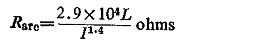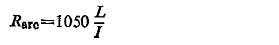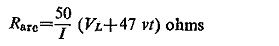## Fault Resistance Calculation:

Fault Resistance Calculation consists of two components, the resistance of the are and the resistance of earth. The second component is present only when it involves an earth-fault. In such a case the resistance of earth would mean the resistance of fault path through the tower, tower footing resistance and earth return.

An approximate value of arc resistance is to be obtained by empirical relations. Warrington gives the expressionwhere

L = length of arc in metres in still air,

I = fault current in amperes.

In the Soviet Union the expression used isL will initially be equal to conductor spacing in the case of phase-faults and distance from conductor to tower in ease of earth faults. With cross wind, when there is a time delay in fault clearance such as in zones 2 and 3, the arc is extended considerably, and the resistance is increased.

For zone 1 where the tripping is instantaneous, the effect of arc resistance is small and may be neglected except on very short feeders; but for zones 2 or 3 high velocity winds may cause the relay to underreach seriously.

When both wind and time are involved, Warrington’s formula for arc resistance iswhere

VL = nominal system interphase voltage, KV

v = wind velocity in km per hour

t = time in second

I = fault current in amperes.

Scroll to Top